Open In App
Related Articles
• CBSE Class 9 Maths Revision Notes
• CBSE Class 9 Maths Formulas
• NCERT Solutions for Class 9 Maths
• RD Sharma Class 9 Solutions

# Circle

In maths, a circle is a 2-D closed shape. It is the basic shape that we observed a lot in our daily life. In maths, a circle is defined as a curve in which all the points on the curve are equal distances from a fixed point. The fixed point is called the centre of the circle and the fixed distance is the radius of the circle. We came across various objects that resemble the circle in real life such as coins, CDs, DVDs, Bangles, etc all resemble circular shapes.

The circle has various formulas including the area of circle and the circumference of circle. Area of the circle is defined as the space occupied inside the boundary of the circle that the circumference of the circle is the length of the boundary of the circle.

Let us more about what is a circle, circle formulas, and important terms along with examples and FAQs in detail in this article.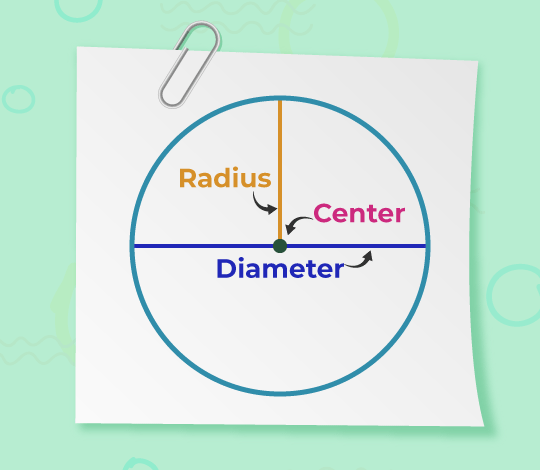Table of Content

## What is Circle in maths?

A circle is a two-dimensional closed figure formed by joining all the points which are at a fixed distance from a fixed point. It is also defined as the locus of the point which is always at a constant distance from a fixed point. The fixed point is called the origin or the centre of the circle and the fixed distance of the points from the origin is called the radius of the circle. We can have an infinite radius of a circle. All the lines passing through the centre of the circle divide the circle into two equal parts that are mirror images of each other. The equation of the circle in the cartesian plane with centre at (h, k) and radius r is,

(x-h)2 + (y-k)2 = r2

### Definition of Circle:

A circle is a two-dimensional geometric shape that consists of all points in a plane that are equidistant (at the same distance) from a fixed point called the center. The distance from the center to any point on the circle is called the radius of the circle

## Circle Shaped Objects

Various objects that we observe in real life are circular shapes. Some examples of circular-shaped objects are,

• Chapattis
• Coins
• Wheels
• Rings
• Buttons
• CDs / DVDs
• Bangles
• Plates

Apart from these many more objects from day-to-day life resembles circular objects. The image added below show some real example of the circle,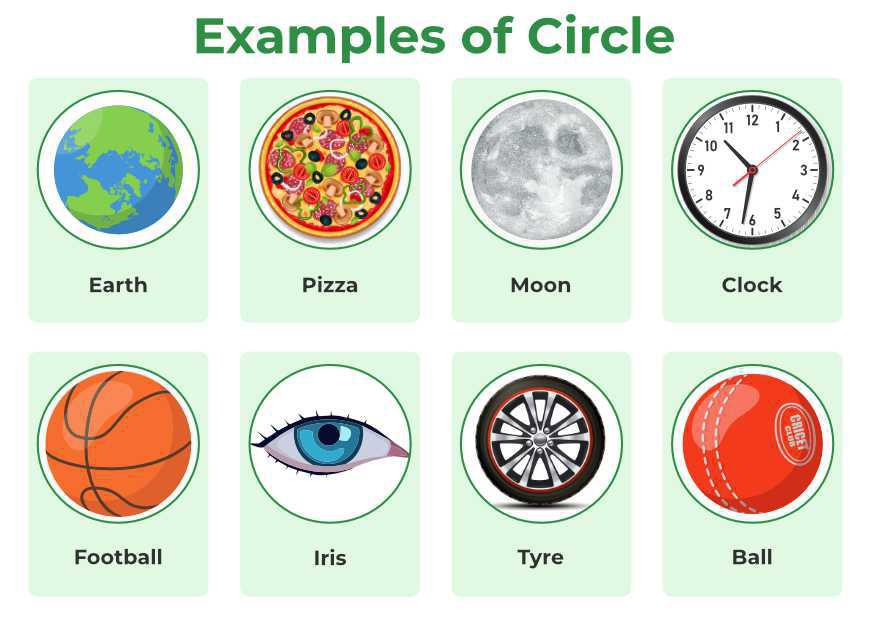## How to Draw a Circle?

Drawing a circle is the most basic construction problem that is taught to students. Construction a circle requires some basic tools such as,

• Pencil
• Compass
• Scale or Ruler

To draw a circle follow the steps below,

Step 1: Attach the pencil to the compass and make sure the tip of the compass and pencil are aligned properly.

Step 2: Mark a point on the paper and call it the centre of the circle.

Step 3: Measure the radius of the circle using the ruler and adjust the compass accordingly.

Step 4: Draw the circle using the compass from the centre point marked in Step 2.

## Parts of a Circle

A circle has various parts and some of the important parts of the circle that are used to study the properties and theorems of the circle are,

Centre of Circle: It is defined as the point from which any point on the circumference of the circle is at a fixed distance.

Radius of Circle: The distance between any point on the circumference of the circle and the fixed point called the centre is called the radius of the circle. It is denoted using the letter ‘r’. A circle can have an infinite radius.

Diameter of Circle: We define the diameter of the circle as the straight line passing through the centre of the circle and connecting any two points on the boundary of the circle. It is denoted by the letter ‘D’. The relation between the radius and the centre is,

D = 2r

Chord of a Circle: Any line which touches the circle at two points on its circumference is the chord of the circle. The diameter is also a chord of the circle. The longest chord of the circle is the diameter of the circle.

Tangent: A line that touches the circle only at one point on its circumference is called the tangent of the circle. It is always perpendicular to the radius of the circle at the point of tangency.

Secant: A line intersecting the circumference of the circle is called the secant on the circle. We also call it the extended chord.

Arc of a Circle: Any portion of the circumference of the circle is called the arc of the circle.

Segment in a Circle: The chord divides the circle into two parts and each part is called the segment of the circle. There are two segments formed by a chord that is,

• Major Segment.
• Minor Segment

Sector of a Circle: The area between two radii and the corresponding arc in a circle is called the sector of the circle. There are two types of sectors

• Major Sector
• Minor Sector

The image added below shows the parts of a circle.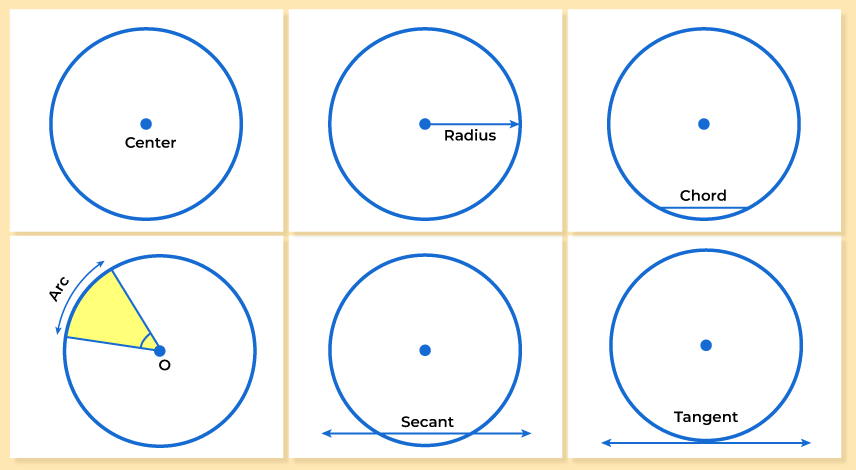The fixed point is named the centre of the circle and therefore the fixed distance is named the radius of the circle. The constant distance between any point on the circle and its centre is called the radius. Note that the line segment joining the centre and any point on the circle is additionally called a radius of the circle.

r = D/2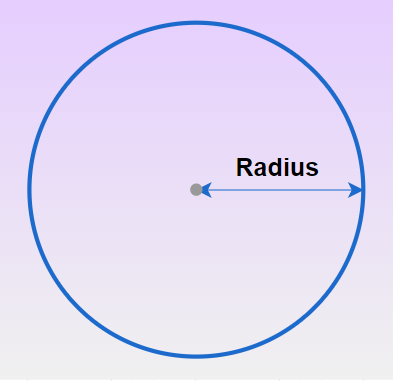### Diameter of Circle (D)

A chord passing through the centre of the circle is called the diameter of the circle. Two radii at 180 degrees angle are the diameter of the circle. Diameter is the largest chord of the circle and every diameter has an equivalent length, which is adequate to twice the radius.

D = 2r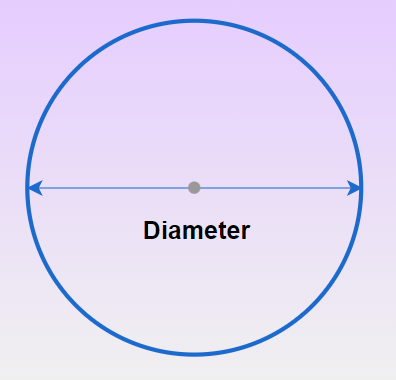## Interior and Exterior of Circle

If we draw a circle it divides the 2-D plane into three parts which are,

• Inside the Circle: A point lying in the plane of the circle such that its distance from its centre is less than the radius of the circle is known as the interior point.
• On the Circle: Points lying in the plane of the circle such that its distance from its centre is equal to the radius of a circle. In simple words, a set of points lying on the circle are points on the circumference of a circle.
• Outside the Circle: Points lying in the plane of the circle such that its distance from its centre is greater than the radius of the circle are exterior points.

## Circle Formulas

There are various formulas related to the circle. Let the radius of the circle is ‘r’ then some of the important formulas related to the circle are,

Parts

Formulae

Area of Circleπr2
Circumference of Circle2πr
Length of Arc

θ × r

where,
‘θ’ is the angle subtended by the arc in radian
‘r’ is the radius of the arc

Area of Sector of Circle

(θ × r2) / 2

where,
‘θ’ is the angle subtended by the arc in radian
‘r’ is the radius of the arc

Length of Chord

2 r sin(θ/2)

where,

Area of Segment

r2(θ − sinθ)/2

where,

The value of π is taken to be 3.14 or 22/7.

## Properties of Circle

A circle is the most common 2-D shape and some of the properties of the circle are,

• Circle with the same radii are called the congruent circle.
• Equal chords are equidistant from the centre of the circle.
• Equidistant chords from the centre of the circle are always equal.
• The perpendicular drawn from the centre of the circle to the chord always bisects the chord.
• We can draw two tangents from an external point to a circle.
• Tangents drawn from the endpoints of the diameter are always parallel to each other.

## Area of a Circle Proof

We can easily prove the formula for the area of the circle using the area of the triangle formula. For this first, we have to draw various concentric circles inside the given circle. Then open all the concentric circles to form a right-angled triangle.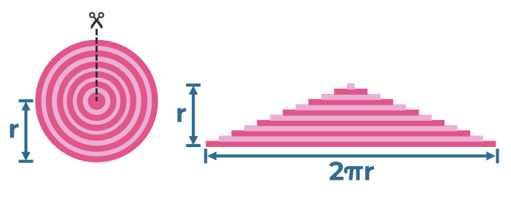If the radius of the given circle is r, then the outer circle would form the base of the right triangle having length 2πr.

The height of the triangle is ‘r’

The area of the right-angled triangle so formed is equal to the area of a circle.

Area of a circle = Area of triangle = (1/2) × base × height

= (1/2) × 2π r  × r

Therefore,

Area of a Circle = πr2

Related Resources:

## Solved Examples on Circles

Example 1: If the diameter of a circle is 142.8 mm, then what is the radius of the circle?

Solution:

Diameter = 142.8 mm

By Formula,

= 71.4 mm

Thus, the radius of the circle is 71.4 mm

Example 2: The distance around a park is 21.98 yd. What is the radius of the park?

Solution:

Circumference of the Park = 21.98 yd

We know that,

= 3.5

Thus, the radius of the circle is 3.5 yd

Example 3: The inner circumference of a circular track is 440 m, and the track is 14 m wide. Calculate the cost of levelling the track at 25 rupees/m2.

Solution:

Let the radius of the inner circle be r m.

Now,

Inner Circumference = 440 m

2πr = 440

2 × 22/7 × r = 440

r = 440 × 744

Width of track = 14 m

= (70 + 14) = 84 m

Area of the Track = π(R2− r2)

= π (842 – 702)

= 22/7 × (7056 – 4900)

= 6776 m2

Cost of levelling at 25 rupees per square meter =  6676 × 25
= 169400 Rupees

Thus, the cost of leveling the track is 169400 Rupees

Example 4: Find the length of the chord of a circle where the radius is 7 cm and the perpendicular distance from the chord to the centre is 4 cm.

Solution:

Given,

Distance of the chord to the centre, d = 3 cm

Chord length = 2√(r2 – d2

= 2√(82 – 32

= 2√(64 – 9)

= 2√55

= 2 × 7.416

Chord length = 14.83 cm

Thus, the length of the chord is 14.83 cm

Example 5: If the radius of a circle is 5 cm and the measure of the angle of the arc is 110˚, what is the length of the arc?

Solution:

Arc Length = (2 × π × r) × angle / 360°

= 2 × 3.14 × 5 × 110/360°

= 9.6 cm

Thus, the length of the arc is 9.6 cm.

Example 6: If the area of a sector with a radius of 6 cm is 35.4 cm2. Calculate the angle of the sector.

Solution:

Area of Sector = Angle/360°  × (π × r × r)

Angle/360° × (π × 6 × 6) = 35.4

Angle = (35.4/36π) × 360°

= 112.67°

Thus, the angle of the sector is, 112.67°

## FAQs on Circles

### 1. What is a Circle in Geometry?

We define a circle in geometry as the locus of the point which is always at a fixed distance from a fixed point, called the centre of the circle.

### 2. What are the formulas of a Circle?

A circle has various formulas but the important formulas for the circle are,

• Area of Circle(A) = πr2
• Circumference of Circle(C) = 2πr

### 3. What is the Chord in a Circle?

A line segment joining two points of the circumference of the circle is called the chord of the circle. A chord passing through the centre of the circle is called the radius of the circle.

### 4. What is the Difference between Chord and Secant in a Circle?

A chord is a line segment which joints any two points on the circumference of the circle, whereas the secant is a line joining any two points on the circumference of the circle, it is also called as extended chord.

### 5. What is the Circumference of a Circle?

The length of the boundary of the circle is called the circumference of the circle. It is calculated using the formula,

C = 2πr

### 6. What is the Area of a Circle?

The space occupied inside the circumference of the circle is called Area of the area of the circle. It is calculated using the formula,

A = πr2

### 7. What are the Properties of Circles?

The properties of the circle are,

• It has the infinite number of radii.
• The radius of the circle is always perpendicular to the tangent of the circle.
• Diameter is the longest chord of the circle

### 8. What are Types of Circles in maths?

We can categorize circles into various categories but the important types of circles are

• Concentric Circles: Circles having different radii but the same centre are called concentric circles.
• Congruent Circles: Circles having the same radius but different centres are called congruent circles.